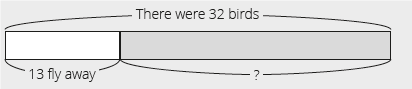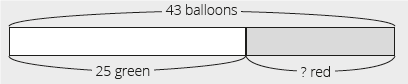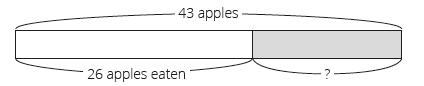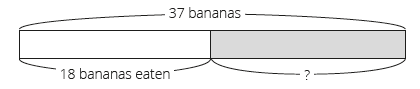# Lesson Notes By Weeks and Term - Primary 2

TERM: 2nd Term

WEEK: 4

CLASS: Primary 2

AGE: 7 years

DURATION: 5 periods of 40 minutes each

DATE:

SUBJECT: Mathematics

SPECIFIC OBJECTIVES: At the end of the lesson, the pupils should be able to

1. Solve subtraction problems with borrowing in context.
2. Write and solve number sentences.

INSTRUCTIONAL TECHNIQUES: Explanation, question and answer, demonstration, practical

INSTRUCTIONAL MATERIALS: Printed tens, bottle tops and place value table per learner, bar diagrams

PERIOD 1: Subtraction with borrowing in context

 PRESENTATION TEACHER’S ACTIVITY PUPIL’S ACTIVITY STEP 1MENTAL MATHS The teacher begins the lesson with some mental calculationsSubtract the following numbers1 14 – 6 =  2 13 – 9 =  3 11 – 7 =  4 12 – 9 =  5 13 – 6 =  6 14 – 7 =7 13 – 8 =8 12 – 6 =9 11 – 8 =10 12 – 3 = Pupils respond and participate STEP 2CONCEPTDEVELOPMENT The teacher• Writes the following word problem on the board. This is an example of a subtraction (change) question. There were 32 birds on the branch.13 of them fly away.How many birds are left? The word problem must be written on three lines as shown above to assist learners to identify the critical information/numbers needed to solve the problem. • Reads the problem.• Lets learners read the problem until they read it fluently.• Underlines the numbers, 32 and 13.• Underlines the question (How many birds are left?) with a wavy line.• Draws the following bar diagram.• Lets learners copy the diagram into their classwork books.• Lets learners write the number sentence.• Lets learners present their number sentence and determine the operation.• Lets the learners solve the number sentence (32 – 13 = 19).• Asks: What is the answer for the word problem? (19 birds left).  CLASS ACTIVITYThe teacherThis second activity is an example of subtraction (combine).• Lets learners solve the problem below by following the same steps as in Activity 1.• Writes the word problem on the board. (Write it on 4 lines as shown below to help learners to identify the problem information.) I have 43 balloons.25 of them are green and the rest are red.How many red balloons do I have? • Draws the following diagram on the board:• The number sentence is 43 – 25 = (18).• The answer is 18 red balloons. Pupils pay attention and participate STEP 3CLASS-WORK This classwork should be done as a class so that you and the learners can discuss and solve the problems together. Write the question and draw a bar diagram. Let learners find the number sentence that expresses the solution to the problem and solve it. You must write the problems on the board (in 3 lines, to show the information clearly) and draw the diagrams with the learners. They will not draw the diagrams by themselves in this lesson (they will do this in a later lesson). Use the diagrams to solve the problem and check your answer using the column method. 1 There are 43 apples.The learners eat 26 apples.How many apples are left?2 I have 52 beads.29 are yellow and the rest are green.How many green beads do I have?Pupils attempt their class work STEP 4HOME-WORK Use the diagram to solve the problem and check your answer using the column method.There are 37 bananas.We eat 18 bananas.How many bananas are left?The pupils writes it in their homework book STEP 5SUMMARY The teacher summarizes by reminding the pupils that in this lesson we have solved real life subtraction problems with borrowing. We used bar diagrams to solve these problems. She marks their class works, makes corrections where necessary and commends them positively

PERIOD 2: Subtraction with borrowing in context

 PRESENTATION TEACHER’S ACTIVITY PUPIL’S ACTIVITY STEP 1MENTAL MATHS The teacher begins the lesson with some mental calculationsCalculate1 15 – 6 =    2 15 – 8 =    3 17 – 9 =    4 16 – 8 =    5 17 – 8 =    6 16 – 7 =7 15 – 7 =8 18 – 9 =9 16 – 9 =10 15 – 9 = Pupils respond and participate STEP 2CONCEPTDEVELOPMENT The teacherThis activity is an example of subtraction (compare).• Lets learners solve the following problem• Writes the following word problem on the board. Nosa has 25 bananas.Belema has 17 bananas.How many more bananas does Nosa have than Belema? • Draws the following diagram on the board:• The number sentence is 25 – 17 = ___ (8).• The answer is Nosa has 8 more bananas than Belema. CLASS ACTIVITYThe teacher• Leaves the bar diagram from activity 1 on the board.• Redraws the 2 bar diagrams from previous lesson• Refers to the 3 different bar diagrams on the board.• Asks: What is common between these three diagrams? (All subtraction problems.)• Asks: What is the difference between the three diagrams? (The diagrams of change and combine are drawn in a row. Meanwhile, compare is drawn in two rows. Drawing two bars helps the learners compare two things as they can see the comparison visually. There are two people/things in combine and compare, while there is only one thing/ person (in this case birds) in change.)NOTE: Learners will not necessarily say “combine”, “compare” or “change” yet. They are expected to find the similar and different aspects between the three types of diagrams such as: I cannot see 13 birds after I subtract, they flew away. But I can see both green and red beads after I subtract.I can also see 25 bananas with Nosa and 17 bananas with Belema after I subtract. The most important thing is that learners can identify that all three types of problems could be solved by subtraction. • You will need to lead this discussion and ask guiding questions. Remember to use the mathematical language in the discussion and use the diagrams to show the answers. Pupils pay attention and participate STEP 3CLASS-WORK Calculate using the column method.a 74 – 56 = __b 65 – 46 = __c 87 – 78 = __d 48 – 39 = __e 35 – 16 = __f 61 – 34 = __ Pupils attempt their class work STEP 4HOME-WORK Calculate using the column method.a 96 – 27 = __b 60 – 21 = __ The pupils writes it in their homework book STEP 5SUMMARY The teacher summarizes by reminding the pupils that in this lesson we have solved subtraction problems with borrowing She marks their class works, makes corrections where necessary and commends them positively

PERIOD 3: Making subtraction number sentences

PRESENTATION

TEACHER’S ACTIVITY

PUPIL’S ACTIVITY

STEP 1

MENTAL MATHS

The teacher begins the lesson with some mental calculations

Subtract the following numbers

1 16 – 9 =

2 13 – 9 =

3 12 – 7 =

4 11 – 8 =

5 17 – 9 =

6 18 – 9 =

7 13 – 8 =

8 14 – 5 =

9 17 – 9 =

10 16 – 8 =

Pupils respond and participate

STEP 2

CONCEPT

DEVELOPMENT

The teacher

• Writes the following number sentence on the board 30 – 3 = __ (27)

• Lets learners solve the problem.

• Asks a learner to come to the board to solve this problem using the column method, showing all the steps and explaining what he/she is doing while they do it.

• Writes the following number sentence: 65 – 38 = __ (27)

• Lets learners solve the problem.

• Asks a learner to come to the board to solve this problem using the column method again, showing all the steps and explaining what he/she is doing while they do it.

Asks: Do you notice anything similar between the two problems? (They both have an answer of 27).

Can you think of another number sentence where the answer is 27? (There are many such sentences.)

Allows the learners to give you examples. Write these on the board and solve them using the column method to check if their examples are correct or not.

CLASS ACTIVITY

The teacher

In this activity, the learners will be finding the missing number.

Writes the following on the board.

 T O 78 13 - 2 9 4 6 0 6 4

O: 13 – 9 = 4

T: 80 – 20 = 60

Discusses with the learners that we need to find the missing numbers – that is the numbers that should be in the blank spaces.

Refers back to column method as an aid.

First, asks: what number should be in the ones place? (13 – 9 = 4)

How many tens did I borrow from the tens place? (1 ten)

I borrowed 1 ten and the number left is 7 tens.

Repeats these steps on following problem.

 T O 56 12 - 3 5 7 2 0 2 7

O: 12 – 7 = 5

T: 50 – 30 = 20

Pupils pay attention and participate

STEP 3

CLASS-WORK

Use the column method to check these answers. Mark each one with a tick or a cross.

1 84 – 15 = 72

2 56 – 19 = 33

3 34 – 18 = 26

4 61 – 7 = 54

Pupils attempt their class work

STEP 4

HOME-WORK

Use the column method to check this answer. Mark it with a tick or a cross.

40 – 3 = 37

The pupils writes it in their homework book

STEP 5

SUMMARY

The teacher summarizes by reminding the pupils that in this lesson we have written and solved subtraction number sentences. We used the column method to check if a subtraction calculation was wrong or correct.

She marks their class works, makes corrections where necessary and commends them positively

PERIOD 4: Consolidation of addition and subtraction

PRESENTATION

TEACHER’S ACTIVITY

PUPIL’S ACTIVITY

STEP 1

MENTAL MATHS

The teacher begins the lesson with some mental calculations

Solve

1 6 + __ = 10

2 __+ 5 =9

3 3 + __ =6

4 3 +__ =9

5 5 +__ =7

6 10 – __ = 8

7 __ – 3 = 6

8 9 – __ = 2

9 10 – __ = 7

10 6 – __ = 6

Pupils respond and participate

STEP 2

CONCEPT

DEVELOPMENT

The teacher

Works through these steps using the following example.

 T O __ 4 + 3 ___ 1 2 8 0 9 2

O: 4 + ___ = 12

T: ____ + 30 = 80

 T O ___ 7 - 3 __ 9 2 0 2 9

O : 17 - ____ = 9

T: ____ - 30 = 20

CLASS ACTIVITY

The teacher

Writes the following on the board.

a 27 + 19 = 36

b 56 – 17 = 49

Asks: What is wrong with these two calculations? (Check the calculations with the class. Go through all of the steps. Refer back to previous examples if necessary.)

Corrects these with the class.

 T O 2 7 + 1 9 1 6 3 0 4 6

O: 7 + 9 = 16

T : 20 + 10 = 30

 T O 45 16 - 1 7 9 3 0 3 9

O: 16 – 7 = 9

T: 40 -10 = 30

As you write down the steps compare the two calculations.

Asks: What was wrong with a) 27 + 19 = 36? (They forgot to carry 1 ten to the tens place from the ones place.)

What was wrong with b) 56 – 17 = 49? (They forgot to borrow 1 ten from 50 in order to subtract correctly in the ones place.)

Pupils pay attention and participate

STEP 3

CLASS-WORK

The learners will be solving addition and subtraction problems in this activity. They will be deciding if the answer given is correct or incorrect. While they are completing this activity,

walk around and discuss the answers and decisions with the learners. In this way, you are checking for understanding.

Check these answers. Mark each one with a tick or a cross.

a 36 + 27 = 62

b 55 + 29 = 84

c 54 + 37 = 99

d 56 – 17 = 39

e 46 – 28 = 16

f 68 – 19 = 49

Pupils attempt their class work

STEP 4

HOME-WORK

Check this answer. Mark it with a tick or a cross.

1 73 – 25 = 48 (correct)

The pupils writes it in their homework book

STEP 5

SUMMARY

The teacher summarizes by reminding the pupils that in this lesson we have found missing numbers, explained what is wrong with a calculation and

checked whether a calculation is correct or wrong.

She marks their class works, makes corrections where necessary and commends them positively

PERIOD 5: Weekly Test/consolidations

TEACHER’S ACTIVITY: The teacher revises all the concepts treated from period 1-4 and gives the pupils follow through exercises, quiz and tests. She marks the exercises, makes corrections and commends the pupils positively.

PUPIL’S ACTIVITY: The pupils work on the worksheets and exercises give by the teacher individually

CONSOLIDATION

Solve the following. Write a number sentence and use the bar diagram to show your answer.

1 There were 26 children in the park.

14 children left the park.

How many children are left?2 Nosa has 27 oranges.

Belema has 17 oranges.

How many more oranges does Nosa have than Belema?3 Fill in the missing numbers

 T O ___ 3 - 2 ___ 6 3 0 3 6

O : 13 - ___ = 6

T : ____ - 20 = 30

4 Check this answer. Mark it with a tick or a cross.

58 – 29 = 39